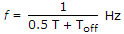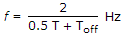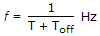# Electronics and Communication Engineering - Power Electronics

### Exercise :: Power Electronics - Section 6

6.

A single phase half wave rectifier is feeding a resistive load. As firing angle is increased

 A. dc output voltage and rms output voltage decrease in the same proportion B. dc output voltage and rms output voltage decrease C. dc output voltage and rms output voltage increase D. dc output voltage and rms output voltage increase in the same proportion

Explanation:

No answer description available for this question. Let us discuss.

7.

A cycloconverter for single phase operation has

 A. one positive group and one negative group converters B. two positive group and two negative group converters C. three positive group and three negative group converters D. any of the above

Explanation:

No answer description available for this question. Let us discuss.

8.

A chopper is a high efficiency circuit.

 A. True B. False

Explanation:

No answer description available for this question. Let us discuss.

9.

The difference between resonant commutation and self commutation is

 A. both these methods are based on entirely different principles B. in resonant commutation, the commutating elements carry load current but in self commutation, the commutating elements do not carry load current C. resonant commutation uses L and C but self commutation uses only capacitor D. none of the above

Explanation:

No answer description available for this question. Let us discuss.

10.

If 0.5 T is the time period of oscillations and Toff is the time between turn off of one thyristor and turn on of second thyristor, the frequency of output f of a series inverter is

 A.B.C.D.